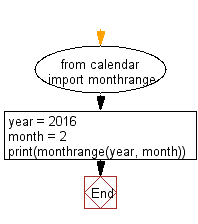﻿ Python: Get the number of days of a given month and year - w3resource

# Python: Get the number of days of a given month and year

## Python Datetime: Exercise-22 with Solution

Write a Python program to get the number of days in a given month and year.

Sample Solution:

Python Code:

``````from calendar import monthrange
year = 2016
month = 2
print(monthrange(year, month))
```
```

Sample Output:

```(0, 29)
```

Flowchart:Python Code Editor: